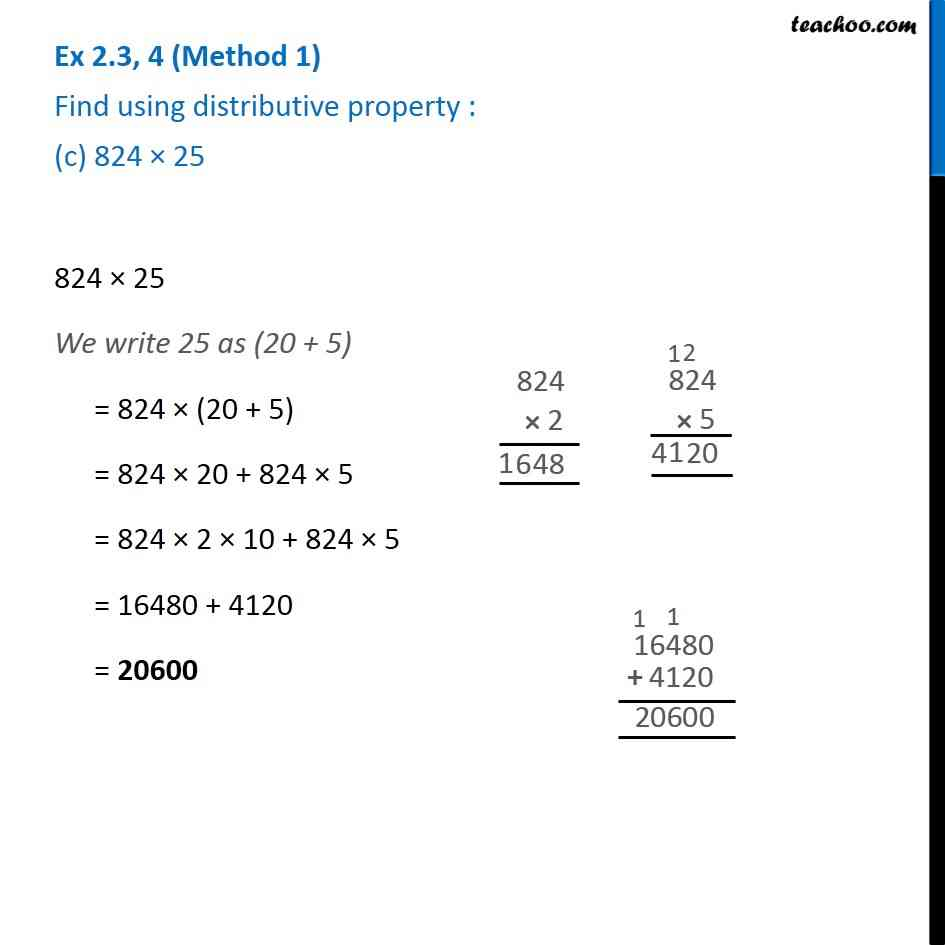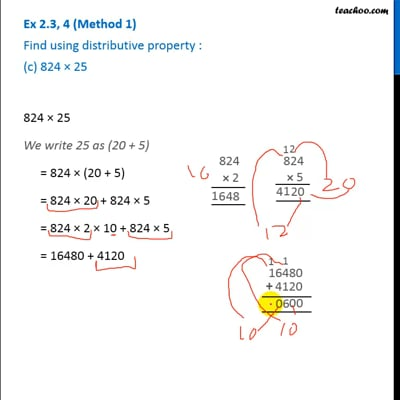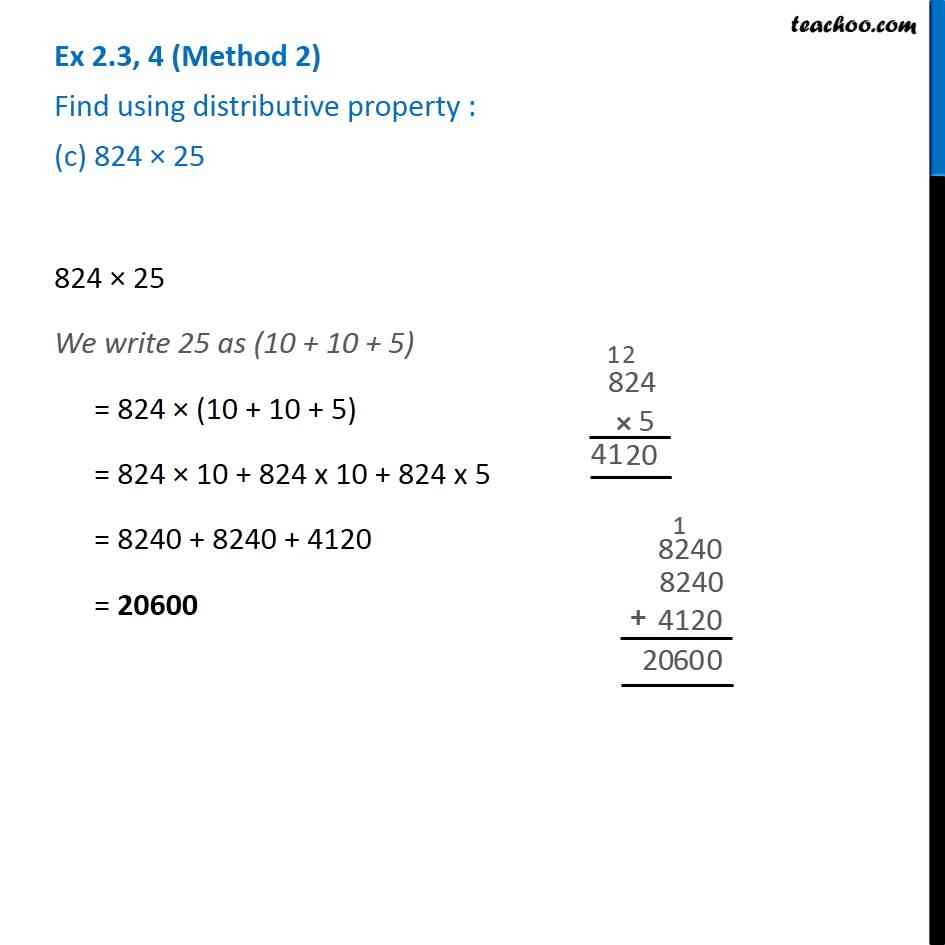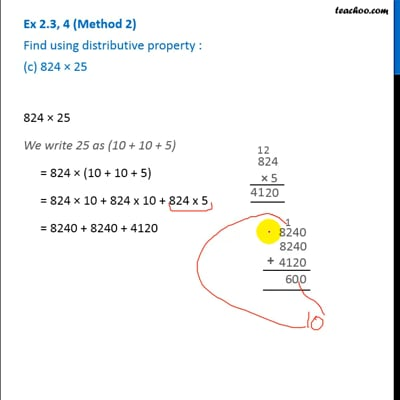Ex 2.3

Chapter 2 Class 6 Whole Numbers
Serial order wiseThis video is only available for Teachoo black usersThis video is only available for Teachoo black users

Introducing your new favourite teacher - Teachoo Black, at only ₹83 per month

### Transcript

Ex 2.3, 4 Find using distributive property : (c) 824 × 25824 × 25 = 824 × (20 + 5) = 824 × 20 + 824 × 5 = 824 × 2 × 10 + 824 × 5 = 16480 + 4120 = 20600 Ex 2.3, 4 (2nd method ) Find using distributive property : (c) 824 × 25824 × 25 = 824 × (10 + 10 + 5) = 824 × 10 + 824 x 10 + 824 x 5 = 8240 + 8240 + 4120 = 20600 Ex 2.3, 4 (2nd method ) Find using distributive property : (c) 824 × 25824 × 25 = 824 × (10 + 10 + 5) = 824 × 10 + 824 x 10 + 824 x 5 = 8240 + 8240 + 4120 = 20600 Ex 2.3, 4 (3rd method ) Find using distributive property : (c) 824 × 25824 × 25 = 824 × 100/4 = 824/4 × 100 = 206 × 100 = 20600